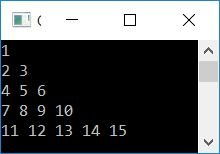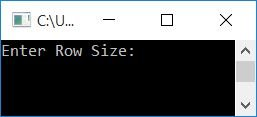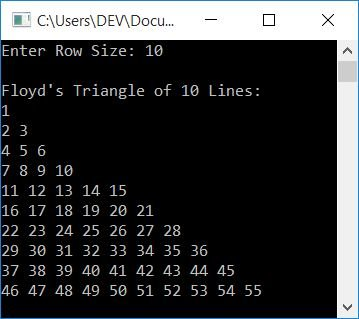# C++ Program to Print Floyd's Triangle

In this article, you will learn how to use a C++ program to print a Floyd's Triangle of the default and user-specified size. Here is the list of programs:

Before creating these programs, let's first understand what a Floyd's Triangle looks like.

### What is Floyd's Triangle?

Floyd's triangle is a right-angled triangle formed by natural numbers, as shown here:

```1
2 3
4 5 6
7 8 9 10
11 12 13 14 15```

## Print Floyd's Triangle in C++

The program given below prints Floyd's triangle of five lines or rows. The question is, "Write a program in C++ to print Floyd's Triangle." Here is its answer:

```#include<iostream>
using namespace std;
int main()
{
int i, j, num=1;
for(i=0; i<5; i++)
{
for(j=0; j<=i; j++)
{
cout<<num<<" ";
num++;
}
cout<<endl;
}
return 0;
}```

This program was built and runs under the Code::Blocks IDE. Here is its sample output:### In C++, print Floyd's Triangle of the Given Size

This program allows the user to define the size of Floyd's triangle. That is, up to the number of lines or rows that Floyd's triangle must expand. Let's have a look at the program:

```#include<iostream>
using namespace std;
int main()
{
int i, j, num=1, rowSize;
cout<<"Enter Row Size: ";
cin>>rowSize;
cout<<"\nFloyd's Triangle of "<<rowSize<<" Lines:\n";
for(i=0; i<rowSize; i++)
{
for(j=0; j<=i; j++)
{
cout<<num<<" ";
num++;
}
cout<<endl;
}
return 0;
}```

Here is its sample run. This is the initial output:Now supply the input, say 10, to print Floyd's triangle of 10 lines as shown in the snapshot given below:#### The same program in different languages

C++ Quiz

« Previous Program Next Program »